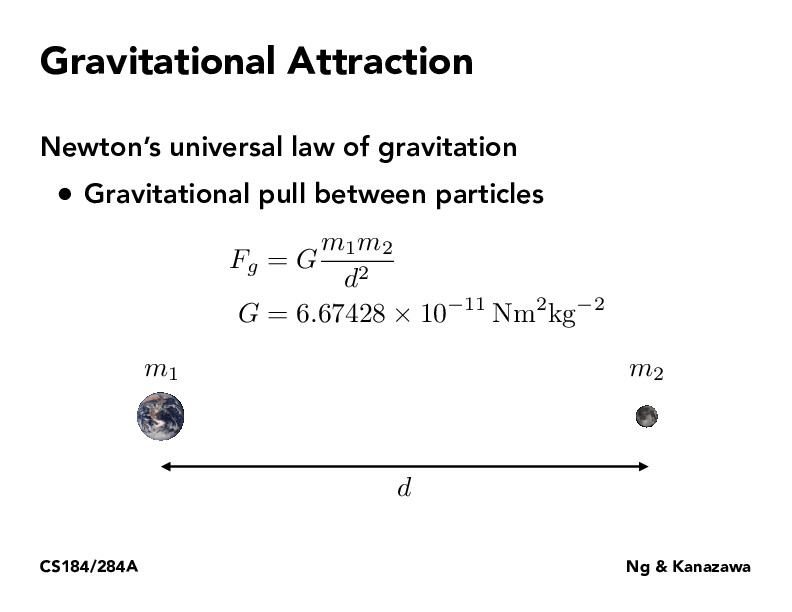Lecture 18: Introduction to Physical Simulation (46)yuhany1024

We can use this equation to estimate the gravity acceleration g: mg= GmM/d^2, where M is the mass of the earth and d is about the radius of the earth.

You must be enrolled in the course to comment